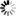# The University of Dodoma Library Catalog

Normal view MARC view ISBD view

# CRC handbook of Lie group analysis of differential equations: Symmetries exact solutions and conservation laws / edited by N.H. Ibragimov.

Material type:TextPublisher: Boca Raton : CRC Press, c1994-c1996Description: 1 v. : ill. ; 25 cm. + 1 computer disk (3 1/2 in.).ISBN: 0849344883 (v. 1); 0849328640 (v. 2 : acidfree); 0849394198 (v. 3 : acidfree).DDC classification: 515/.35 Online resources: Publisher description
Contents:
v. 1. Symmetries, exact solutions, and conservation laws / W.F. Ames ... [et al.] -- v. 2. Applications in engineering and physical sciences / A.V. Aksenov ... [et al.] -- v. 3. New trends in theoretical developments and computational methods / R.L. Anderson ... [et al.].
Tags from this library: No tags from this library for this title.Average rating: 0.0 (0 votes)
Item type Current location Call number Copy number Status Date due BarcodeBooks Library, College of Natural and Mathematical Sciences (CNMS)
Special Reserve
QA372 .C73 1994 (Browse shelf) 1-1 Available 8372
##### Browsing Library, College of Natural and Mathematical Sciences (CNMS) Shelves , Shelving location: Special Reserve Close shelf browser
 No cover image available No cover image available QA371.5.D37 D53 1996 Differential equations with Maple / QA372 .C59 2012 An introduction to ordinary differential equations. QA372 .C6417 2003 Differential equations with boundary value problems : QA372 .C73 1994 CRC handbook of Lie group analysis of differential equations: Symmetries exact solutions and conservation laws / QA372 .E34 2001 Differential equations and linear algebra / QA372 .G728 2006 Numerical solution of ordinary differential equations : QA372 .R77 2004 An introduction to ordinary differential equations /

Vol. 1

Includes bibliographical references and indexes.

v. 1. Symmetries, exact solutions, and conservation laws / W.F. Ames ... [et al.] -- v. 2. Applications in engineering and physical sciences / A.V. Aksenov ... [et al.] -- v. 3. New trends in theoretical developments and computational methods / R.L. Anderson ... [et al.].

There are no comments for this item.